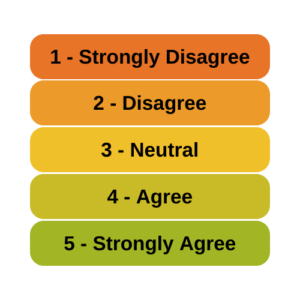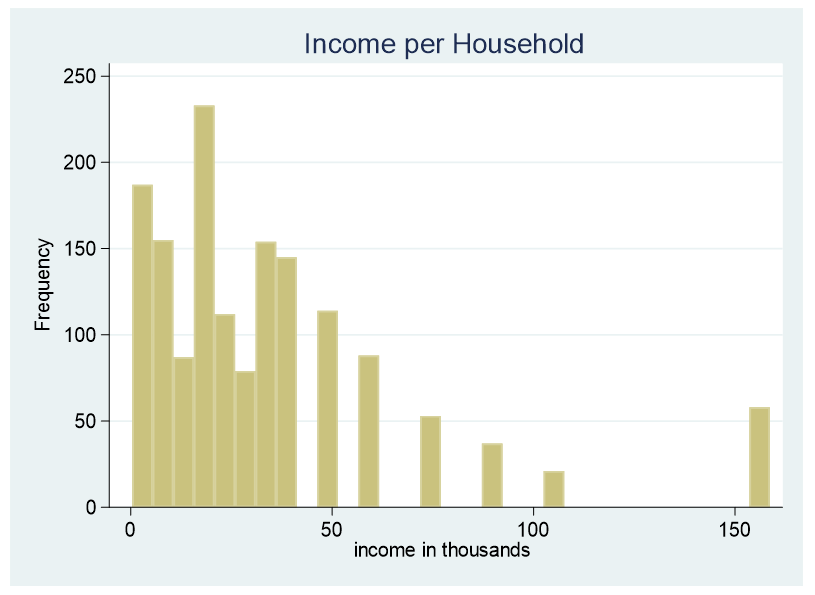# continuous variable

### Can Likert Scale Data ever be Continuous?

January 19th, 2023 by

A very common question is whether it is legitimate to use Likert scale data in parametric statistical procedures thatrequire interval data, such as Linear Regression, ANOVA, and Factor Analysis.

A typical Likert scale item has 5 to 11 points that indicate the degree of something. For example, it could measure agreement with a statement, such as 1=Strongly Disagree to 5=Strongly Agree. It can be a 1 to 5 scale, 0 to 10, etc. (more…)

### A Strategy for Converting a Continuous to a Categorical Predictor

February 18th, 2019 by

At times it is necessary to convert a continuous predictor into a categorical predictor.  For example, income per household is shown below.This data is censored, all family income above \$155,000 is stated as \$155,000. A further explanation about censored and truncated data can be found here. It would be incorrect to use this variable as a continuous predictor due to its censoring.

### A Useful Graph for Interpreting Interactions between Continuous Variables

February 11th, 2019 by

What’s a good method for interpreting the results of a model with two continuous predictors and their interaction?Let’s start by looking at a model without an interaction.  In the model below, we regress a subject’s hip size on their weight and height. Height and weight are centered at their means.

### Understanding Interactions Between Categorical and Continuous Variables in Linear Regression

May 14th, 2018 by

We’ve looked at the interaction effect between two categorical variables. Now let’s make things a little more interesting, shall we?

What if our predictors of interest, say, are a categorical and a continuous variable? How do we interpret the interaction between the two? (more…)

### Differences Between the Normal and Poisson Distributions

December 23rd, 2016 by

The normal distribution is so ubiquitous in statistics that those of us who use a lot of statistics tend to forget it’s not always so common in actual data.

And since the normal distribution is continuous, many people describe all numerical variables as continuous. I get it: I’m guilty of using those terms interchangeably, too, but they’re not exactly the same.

Numerical variables can be either continuous or discrete.

The difference? Continuous variables can take any number within a range. Discrete variables can only be whole numbers.

So 3.04873658 is a possible value of a continuous variable, but not discrete.

Count variables, as the name implies, are frequencies of some event or state. Number of arrests, fish (more…)

### The Impact of Removing the Constant from a Regression Model: The Categorical Case

December 9th, 2016 byIn a simple linear regression model, how the constant (a.k.a., intercept) is interpreted depends upon the type of predictor (independent) variable.

If the predictor is categorical and dummy-coded, the constant is the mean value of the outcome variable for the reference category only. If the predictor variable is continuous, the constant equals the predicted value of the outcome variable when the predictor variable equals zero.

### Removing the Constant When the Predictor Is Categorical

When your predictor variable X is categorical, the results are logical. Let’s look at an example. (more…)